Search

# Subspace clustering

Updated: Jun 22, 2019

### Challenges in high dimensional spaces

This post addresses the following questions:

1. What are the challenges of working with high dimensional data?

2. What is subspace clustering?

3. How to implement a subspace clustering algorithm in pythonHigh dimensional data consists in input having from a few dozen to many thousands of features (or dimensions). This is a context typically encountered for instance in bioinformatics (all sorts of sequencing data) or in NLP where the size of the vocabulary if very high. High dimensional data is challenging because:

- it makes the visualization and thus understanding of the input difficult, it often requires applying a dimensionality reduction technique beforehand. It leads to the ‘curse of dimensionality’ which means that the complete enumeration of all subspaces becomes intractable with increasing dimensionality

- most underlying clustering techniques depend on the results and the choice of the dimensionality reduction technique

- many dimensions may be irrelevant and can mask existing clusters in noisy data

- one common technique is to perform feature selection (remove irrelevant dimensions) but there are cases when identifying redundant dimensions is not easy

## What is subspace clustering?

Subspace clustering is a technique which finds clusters within different subspaces (a selection of one or more dimensions). The underlying assumption is that we can find valid clusters which are defined by only a subset of dimensions (it is not needed to have the agreement of all N features). For example, if we consider as input patient data observing the gene expression level (we can have more than 20000 features), a cluster of patients suffering from Alzheimer can be found only by looking at the expression data of a subset of 100 genes, or stated differently, the subset exists in 100D. Stated differently, subspace clustering is an extension of traditional N dimensional cluster analysis which allows to simultaneously group features and observations by creating both row and column clusters.

The resulting clusters may be overlapping both in the space of features and observations. Another example is shown in the figures below, taken from the paper. We can notice that points from 2 clusters can be very close which can confuse many traditional clustering algorithms analyzing the entire feature space.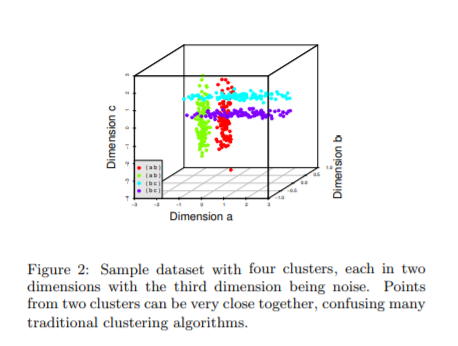Further more, we can see that subspace clustering manages to find a subspace (dimensions a and c) where the expected clusters are easily identifiable.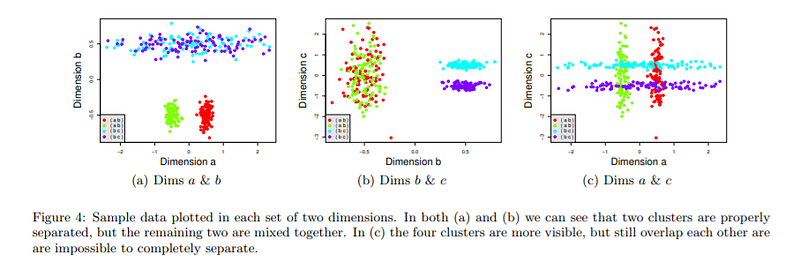## Types of subspace clustering

Based on the search strategy, we can differentiate 2 types of subspace clustering, as shown in the figure below: bottom up approaches start by finding clusters in low dimensional (1 D) spaces and iteratively merging them to process higher dimensional spaces (up to N D). Top down approaches find clusters in the full set of dimensions and evaluate the subspace of each cluster. The figure below, taken from the same paper provides an overview of the most common subspace clustering algorithms.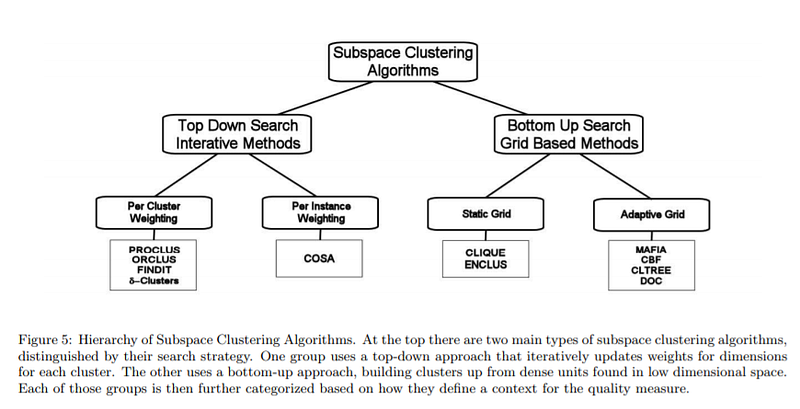## Clique algorithm

In order to better understand subspace clustering, I have implemented the Clique algorithm in python here.

In a nutshell, the algorithm functions as follows: for each dimension (feature) we split the space in nBins(input parameter) and for each bin we compute the histogram (number of counts). We only consider dense units, that is the bins with a count superior to a threshold given as second input parameter. A dense unit is characterized by the following:

- the dimension it belongs to (e.g. feature 1)

- the index (or the position) of the bin (from 0 to nBins)

- the observations lying in the bin

In my implementation I have generated 4 random clusters in a 2D space and I have chosen 8 bins and 2 points as minimal density threshold. The figure below shows the resulting grid applied to the input space.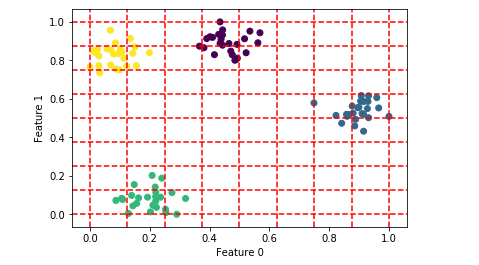The intuition behind the clique algorithm is that clusters existing in a k dimensional space can also be found in k-1. We start from 1D and for each dimension we try to find the dense bins. If 2 or more dense bins are neighbors, we merge them into one bigger bin. This operation can be easily implemented by transforming all existing dense bins into a graph, where an edge is drawn if 2 dense units belong to the same dimension and the difference between their bin index is no more than 1 ( e.g a dense unit corresponding to feature 3 and bin 4 is neighbor for dense units of the same feature and bins 3 and 5). The dense units to be merged can be identified by calculating the connected components on the graph described above.

The result of this merging operation retrieves the following clusters in 1 D (one plot per cluster) for the first dimension: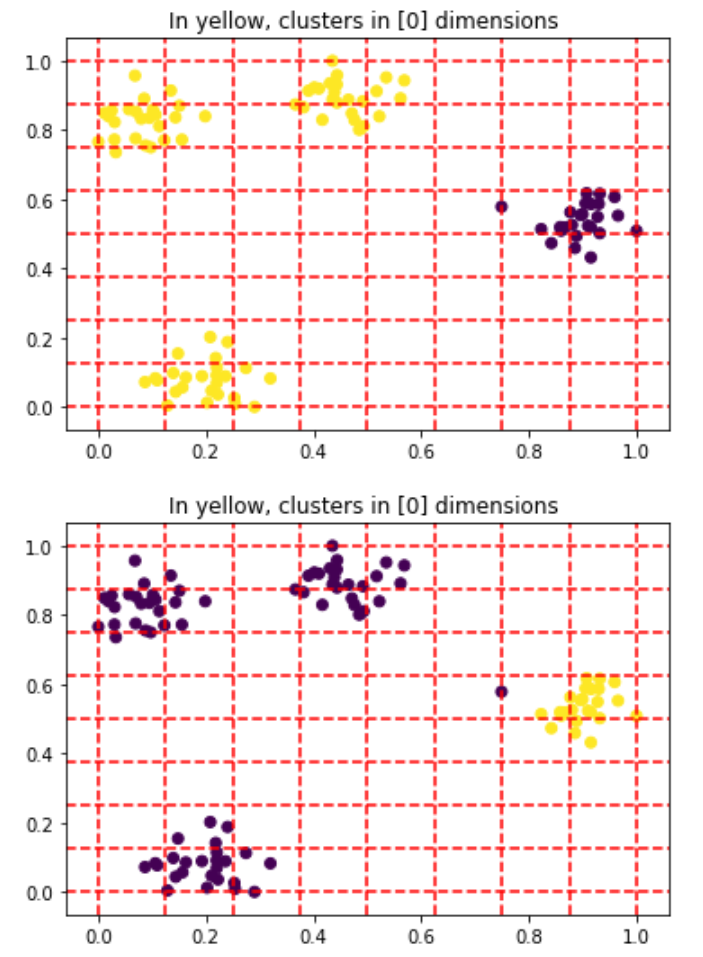and for the second dimension: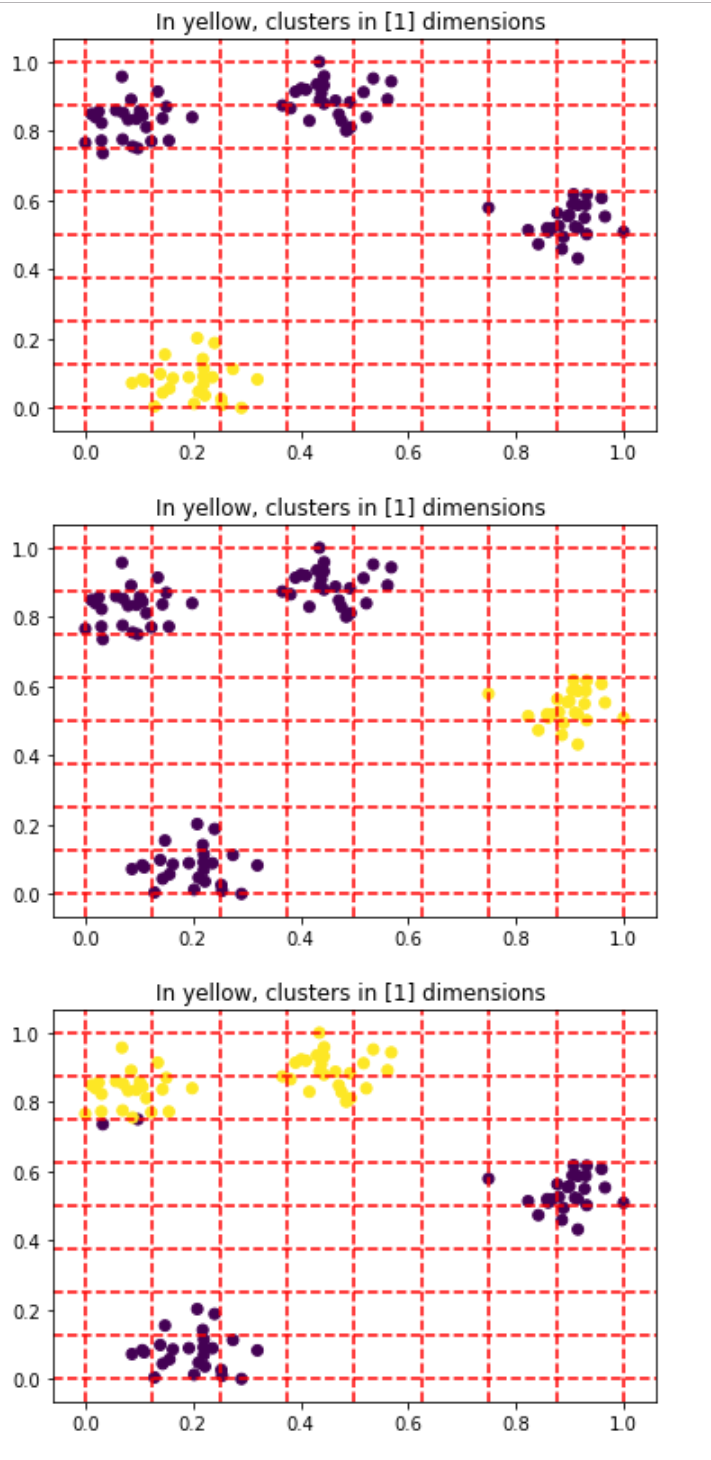Next, we want to calculate all valid clusters in each subspace from 2 to the number of input dimensions. This operation comes down to calculating combinations of dense units in k dimensions and only keeping results having an overlap of dense continuous bins with the size greater than the initial minimal density threshold. Once we calculated the dense units for k-1 dimensions we can extend to k dimensions by computing all combinations of these last k-1 candidates.

Thus, in 2 D we are able to retrieve the clusters shown in the figure below. Note that some points (in purple) outside the 4 clusters because they belong to bins having a density inferior to the arbitrary input of 2.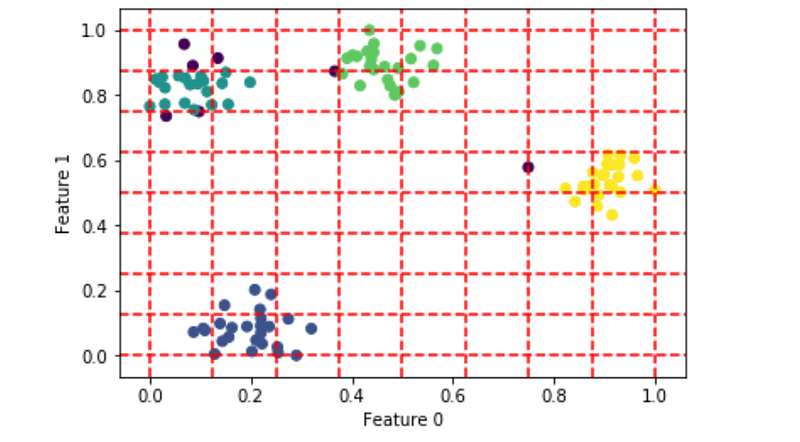Clique clustering has been criticized for its high sensitivity to the input parameters (the number of bins and the minimal density) which can lead to very different results. However, it is an essential algorithm in the family of bottom-up subspace clustering. There are multiple ways to optimize the clique algorithm, for instance by using a density adaptive grid as proposed in the MAFIA algorithm.

## References

Clique paper

Mafia algorithm

Comparative study of subspace clustering methods

Presentation of subspace clustering methods

See All